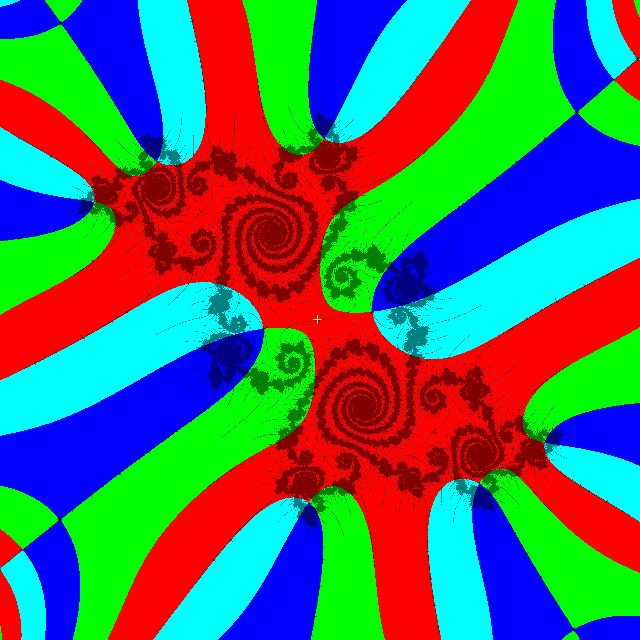# mathr / blog / #

## Periodicity scan revisited

Wolf Jung's Mandel's "algorithm 9" allows locating zeroes of the iterated polynomial at a certain period where 4 colours meet. But I wanted to find the zeroes for lots of periods all at once. Previously I did this in a way that didn't scale efficiently to deep zooms, so I adapted the "algorithm 9" technique. Not implemented yet is the extension of this code to use perturbation techniques for deep zooms, but it should be perfectly possible.The first thing to do is initialize the array of $$c$$ values, here I use my mandelbrot-graphics library as the support code (not shown here) uses it for imaging:

void initialize_cs(int m, int n, m_d_transform *t, double _Complex *cs)
{
#pragma omp parallel for
for (int j = 0; j < n; ++j)
{
for (int i = 0; i < m; ++i)
{
double _Complex c = i + I * j;
double _Complex dc = 1;
m_d_transform_forward(t, &c, &dc);
int k = i + j * m;
cs[k] = c;
}
}
}

Then in the iteration step, calculate a flag for which quadrant the $$z$$ iterate is in. This is set as a bit mask, so ORing many masks together corresponds to set union:

void step_zs(int mn, char *qs, double _Complex *zs, const double _Complex *cs)
{
#pragma omp parallel for
for (int i = 0; i < mn; ++i)
{
double _Complex c = cs[i];
double _Complex z = zs[i];
// step
z = z * z + c;
char q = 1 << ((creal(z) > 0) | ((cimag(z) > 0) << 1));
// store
zs[i] = z;
qs[i] = q;
}
}

Now the meat of the algorithm: it scans across the data with a 3x3 window, to find where all 4 colours meet in one small square. Then if that happens, check that the 3x3 square has a local minimum at its center, which means that the point found is really near a zero (a proof for that assertion follows immediately from the minimum modulus principle).

int scan_for_zeroes(int m, int n, int ip, int *ops, double _Complex *ocs, const char *qs, const double _Complex *zs, const double _Complex *ics)
{
int o = 0;
// loop over image interior, to avoid tests in inner 3x3 loop
#pragma omp parallel for
for (int j = 1; j < n - 1; ++j)
{
for (int i = 1; i < m - 1; ++i)
{
// find where 4 quadrants meet in 3x3 region
char q = 0;
for (int dj = -1; dj <= 1; ++dj)
{
int jdj = j + dj;
for (int di = -1; di <= 1; ++di)
{
int idi = i + di;
int kdk = idi + jdj * m;
q |= qs[kdk];
}
}
if (q == 0xF)
{
// 4 quadrants meet, check for local minimum at center
double minmz = 1.0/0.0;
for (int dj = -1; dj <= 1; ++dj)
{
int jdj = j + dj;
for (int di = -1; di <= 1; ++di)
{
int idi = i + di;
int kdk = idi + jdj * m;
double mz = cabs(zs[kdk]);
minmz = mz < minmz ? mz : minmz;
}
}
int k = i + j * m;
double mz = cabs(zs[k]);
if (mz <= minmz && minmz < 1.0/0.0)
{
// we found a probable zero, output it
double _Complex ic = ics[k];
int out;
#pragma omp atomic capture
out = o++;
ops[out] = ip;
ocs[out] = ic;
}
}
}
}
return o;
}

To be safe, the output arrays should be sized at least the desired number of elements plus the number of pixels in the image (which is the maximum number that can be output in one pass). Most of the extra space will be unused by the time the stopping condition (enough space left) is reached.

An earlier version was several times slower, partly due to caching cabs() calls for every pixel, though only very few pixels are near a zero at any given iteration.